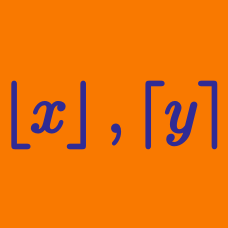Algebra

# Floor and Ceiling Functions: Level 1 Challenges

$\large \lim_{x\to \infty}\dfrac{\lfloor \sqrt x \rfloor^2}{x} = \ ?$

Note: Type -1000 as an answer if you think this limit does not exist.

Find the number of trailing zeros of the number $60!$.

For all $x \ge 0$, and $x \in \mathbb {\text{R}}$, is it true that

$\left \lfloor \sqrt{\lfloor x \rfloor}\right \rfloor = \left \lfloor \sqrt{x} \right \rfloor$

Let $\left\lfloor x \right\rfloor$ denote the greatest integer less that or equal to $x$.

So, Find the sum of all possible solutions of

$\left\lfloor x \right\rfloor+ \left\lfloor 2x \right\rfloor+\left\lfloor 4x \right\rfloor+\left\lfloor 8x \right\rfloor+\left\lfloor 16x \right\rfloor+\left\lfloor 32x \right\rfloor=12345$

Note: If you feel that there are no such possible values of x, type the answer as $0$

If $f(x) = \lceil x^{2}\rceil$, what is the value of $f\left(\sqrt {\sqrt {5}} \right)$? 

Notation: $\lceil \cdot \rceil$ denotes the ceiling function.

×